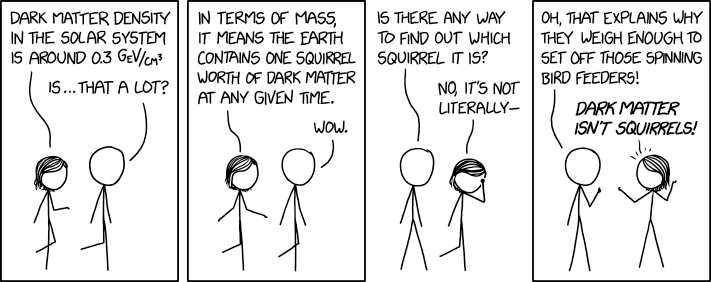One of last week’s xkcd comics was about the local dark matter density.Wait. If only 5% of the universe is ordinary matter and 25% is dark matter (the rest being dark energy), see 1 and 2, then why is there only one dark matter squirrel on earth? This is a good opportunity to play around with the Python package pyveu.

The local dark matter density is $$\rho = (0.3 \pm 0.1)\;\mathrm{GeV} / \mathrm{cm}^3$$, see 3. In this context, masses are measured in $$\mathrm{GeV}$$ because $$E=mc^2$$ and physicists often measure speed in multiplies (or rather fractions) of the speed of light (or in short $$c=1$$). Earth’s radius is $$r=6371\,\mathrm{km}$$. The uncertainty of the radius is negligible compared to the uncertainty on the density. However, for the sake of this example, let’s assume that there is an uncertainty of $$10\,\mathrm{km}$$ on the radius due to the fact that earth is not a perfect sphere.

So let’s define this in pyveu.

from pyveu import Quantity
import math

rho = Quantity("0.3 +- 0.1 GeV / cm^3 / c^2")
radius = Quantity("6371 +- 10 km")

volume = 4 / 3 * math.pi * radius**3

m_squirrel = rho * volume
print(m_squirrel)


If we run this, we get the confirmation

\$ python3 earth.py
(0.58 +- 0.19) kg


that earth contains one squirrel worth of dark matter.

Ok, to be fair, our galaxy is mostly empty space, i.e., empty in terms of ordinary matter. Earth, or our sun, is just an extreme local concentration of ordinary matter. The contribution of dark matter should outweigh the contribution of ordinary matter at larger scales.